Courses

# Atomic Spectra Notes | EduRev

## JEE : Atomic Spectra Notes | EduRev

The document Atomic Spectra Notes | EduRev is a part of the JEE Course Physics Class 12.
All you need of JEE at this link: JEE

All condensed matter (solids, liquids, and dense gases) emit electromagnetic radiation at all temperatures. Also, this radiation has a continuous distribution of several wavelengths with different intensities. This is caused by oscillating atoms and molecules and their interaction with the neighbours. In the early nineteenth century, it was established that each element is associated with a characteristic spectrum of radiation, known as Atomic Spectra. Hence, this suggests an intimate relationship between the internal structure of an atom and the spectrum emitted by it.

Atomic Spectra

When an atomic gas or vapour is excited under low pressure by passing an electric current through it, the spectrum of the emitted radiation has specific wavelengths. It is important to note that, such a spectrum consists of bright lines on a dark background. This is an emission line spectrum. Here is an emission line spectrum of hydrogen gas: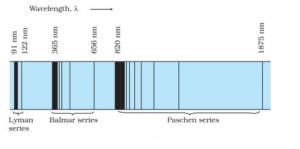Emission lines in the spectrum of hydrogen

The emission line spectra work as a ‘fingerprint’ for identification of the gas. Also, on passing a white light through the gas, the transmitted light shows some dark lines in the spectrum. These lines correspond to those wavelengths that are found in the emission line spectra of the gas. This is the absorption spectrum of the material of the gas.

Spectral Series of Atomic Spectra

Normally, one would expect to find a regular pattern in the frequencies of light emitted by a particular element. Let’s look at hydrogen as an example. Interestingly, at the first glance, it is difficult to find any regularity or order in the atomic spectra. However, on close observation, it can be seen that the spacing between lines within certain sets decreases in a regular manner. Each of these sets is a spectral series.

Five spectral series identified in hydrogen are

1. Balmer Series
2. Lyman Series
3. Paschen Series
4. Brackett Series
5. Pfund Series

Further, let’s look at the Balmer series in detail.

1. Balmer Series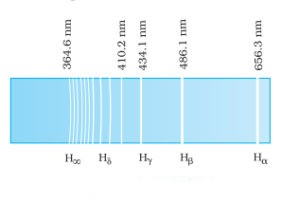Balmer series in the emission spectrum of hydrogen In 1885, when Johann Balmer observed a spectral series in the visible spectrum of hydrogen, he made the following observations:

• The longest wavelength is 656.3 nm
• The second longest wavelength is 486.1 nm
• And the third is 434.1 nm
• Also, as the wavelength decreases the lines appear closer together and weak in intensity
• He found a simple formula for the observed wavelengths: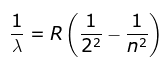where λ is the wavelength
R is the Rydberg's constant and
n can have any integral value.

Further, for n=∞, you can get the limit of the series at a wavelength of 364.6 nm. Also, you can’t see any lines beyond this; only a faint continuous spectrum. Furthermore, like the Balmer’s formula, here are the formulae for the other series:

2. Lyman Series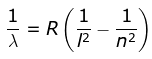where λ is the wavelength
R is the Rydberg's constant and
n can have any integral value.

3. Paschen Series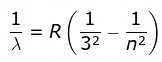where λ is the wavelength
R is the Rydberg's constant and
n can have any integral value

4. Brackett Series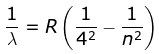where λ is the wavelength
R is the Rydberg's constant and
n can have any integral value

5. Pfund Series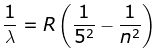where λ is the wavelength
R is the Rydberg's constant and
n can have any integral value

Offer running on EduRev: Apply code STAYHOME200 to get INR 200 off on our premium plan EduRev Infinity!

## Physics Class 12

132 videos|307 docs|166 tests

,

,

,

,

,

,

,

,

,

,

,

,

,

,

,

,

,

,

,

,

,

;